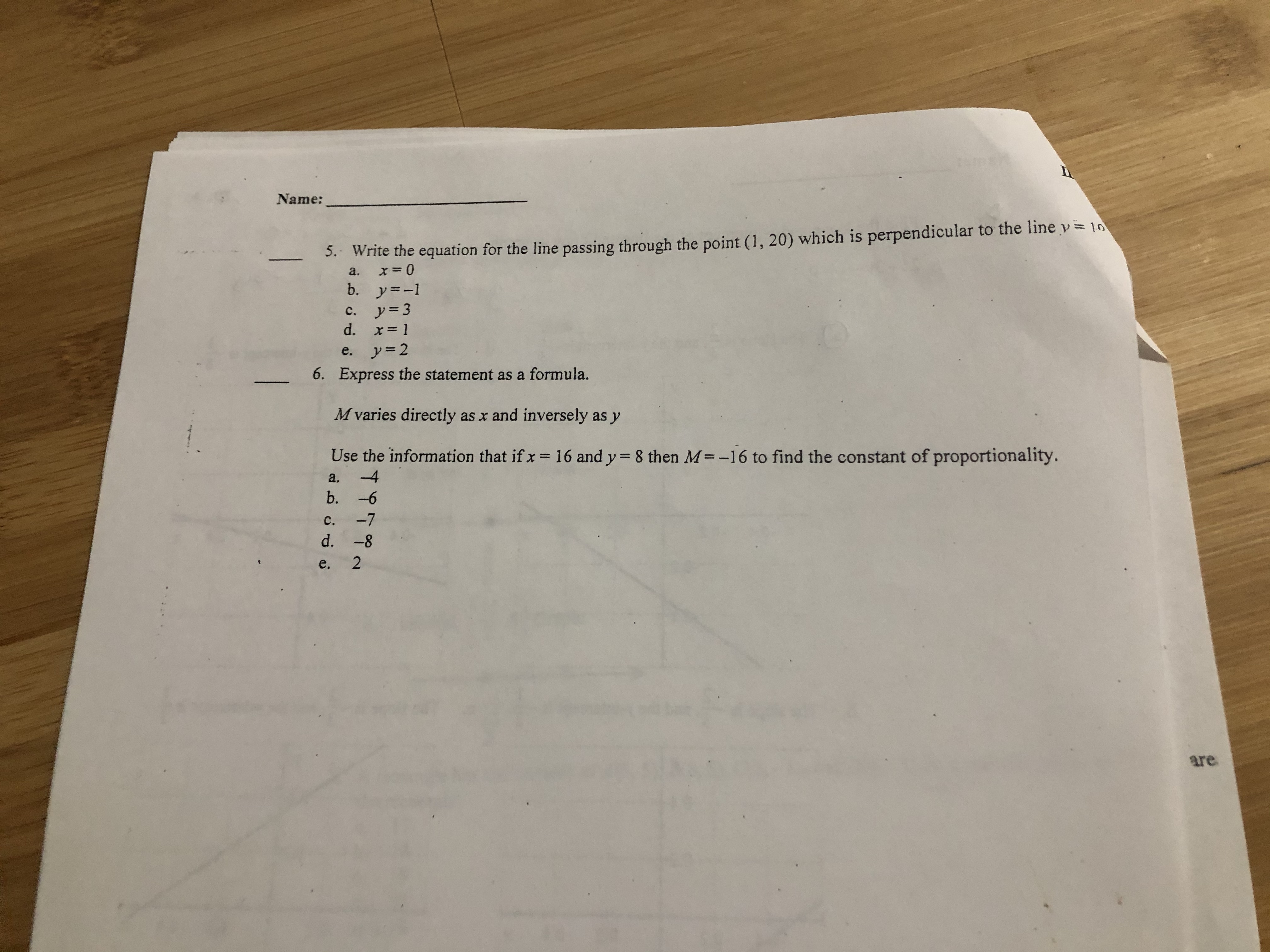# 20Name:5.. Write the equation for the line passing through the point (1, 20) which is perpendicular to the line v= 10a.b. y=-1c. y=3d. x= 1e. y=26. Express the statement as a formula.M varies directly as x and inversely as yUse the information that if x16 and y= 8 then M=-16 to find the constant of proportionality.%3Da.-4b. -6c.-7d. -8e,are

Question
2 viewshelp_outlineImage Transcriptionclose20 Name: 5.. Write the equation for the line passing through the point (1, 20) which is perpendicular to the line v= 10 a. b. y=-1 c. y=3 d. x= 1 e. y=2 6. Express the statement as a formula. M varies directly as x and inversely as y Use the information that if x 16 and y= 8 then M=-16 to find the constant of proportionality. %3D a. -4 b. -6 c. -7 d. -8 e, are fullscreen
check_circle

Step 1

The statement “M varies directly as x and inversely as...

### Want to see the full answer?

See Solution

#### Want to see this answer and more?

Solutions are written by subject experts who are available 24/7. Questions are typically answered within 1 hour.*

See Solution
*Response times may vary by subject and question.
Tagged in

### Relations and Sets